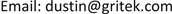1. 概述

2. 试验2.1. 样品

2.2. 清洗设备

SPMC-5.6寸全自动硅片清洗设备。

2.3. 工艺流程

2.4. 实验方案

3. 结果3.1. 表面颗粒水平

3.2. 表面金属水平

Surface metal level of each group of experiment

02 h加活性剂1.5<0.40.5<0.51.30.5<0.21.2<0.4<0.5<0.10.11

08 h加活性剂1.2<0.4<0.5<0.51.9<0.3<0.23.9<0.4<0.5<0.10.12

12 h加活性剂30.510.8<0.8<0.30.94.10.9<0.5<0.1<0.12

18 h加活性剂4.41.8<0.50.5<0.8<0.3<0.25.3<0.4<0.5<0.10.32

24 h加活性剂6.61.9<0.50.5<0.8<0.3<0.24.2<0.4<0.5<0.1<0.11

36 h加活性剂9.93.50.50.7<0.8<0.3<0.24.5<0.4<0.5<0.10.16

4. 总结